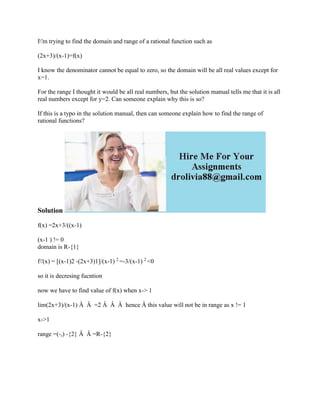Ce diaporama a bien été signalé.
Le téléchargement de votre SlideShare est en cours. ×

# I-'m trying to find the domain and range of a rational function such a.docx

Publicité
Publicité
Publicité
Publicité
Publicité
Publicité
Publicité
Publicité
Publicité
Publicité
PublicitéChargement dans…3
×

## Consultez-les par la suite

1 sur 2 Publicité

# I-'m trying to find the domain and range of a rational function such a.docx

I\'m trying to find the domain and range of a rational function such as

(2x+3)/(x-1)=f(x)

I know the denominator cannot be equal to zero, so the domain will be all real values except for x=1.

For the range I thought it would be all real numbers, but the solution manual tells me that it is all real numbers except for y=2. Can someone explain why this is so?

If this is a typo in the solution manual, then can someone explain how to find the range of rational functions?
Solution
f(x) =2x+3/((x-1)

(x-1 ) != 0
domain is R-{1}

f\'(x) = [(x-1)2 -(2x+3)1]/(x-1) 2 =-3/(x-1) 2 <0
so it is decresing fucntion
now we have to find value of f(x) when x-> 1
lim(2x+3)/(x-1) Â  Â  =2 Â  Â  Â  hence Â this value will not be in range as x != 1
x->1
range =(-,) -{2} Â  Â =R-{2}
.

I\'m trying to find the domain and range of a rational function such as

(2x+3)/(x-1)=f(x)

I know the denominator cannot be equal to zero, so the domain will be all real values except for x=1.

For the range I thought it would be all real numbers, but the solution manual tells me that it is all real numbers except for y=2. Can someone explain why this is so?

If this is a typo in the solution manual, then can someone explain how to find the range of rational functions?
Solution
f(x) =2x+3/((x-1)

(x-1 ) != 0
domain is R-{1}

f\'(x) = [(x-1)2 -(2x+3)1]/(x-1) 2 =-3/(x-1) 2 <0
so it is decresing fucntion
now we have to find value of f(x) when x-> 1
lim(2x+3)/(x-1) Â  Â  =2 Â  Â  Â  hence Â this value will not be in range as x != 1
x->1
range =(-,) -{2} Â  Â =R-{2}
.

Publicité
Publicité

### I-'m trying to find the domain and range of a rational function such a.docx

1. 1. I'm trying to find the domain and range of a rational function such as (2x+3)/(x-1)=f(x) I know the denominator cannot be equal to zero, so the domain will be all real values except for x=1. For the range I thought it would be all real numbers, but the solution manual tells me that it is all real numbers except for y=2. Can someone explain why this is so? If this is a typo in the solution manual, then can someone explain how to find the range of rational functions? Solution f(x) =2x+3/((x-1) (x-1 ) != 0 domain is R-{1} f'(x) = [(x-1)2 -(2x+3)1]/(x-1) 2 =-3/(x-1) 2 <0 so it is decresing fucntion now we have to find value of f(x) when x-> 1 lim(2x+3)/(x-1) Â Â =2 Â Â Â hence Â this value will not be in range as x != 1 x->1 range =(-,) -{2} Â Â =R-{2}